# Solution assignment 07 Exponential functions and graphs

### Assignment 7

Draw the graph of the function: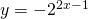What is the formula of the asymptote?

### Solution

First we look at the function: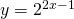This is a function with a shifted graph of: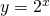We can sketch the graph of this function quite easily. Square its graph, shift it to the right and divide the result by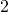. When we mirror this function in the-axis we get the final result. The-axis, i.e.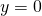is the asymptote of the graph.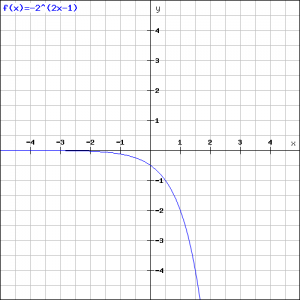0